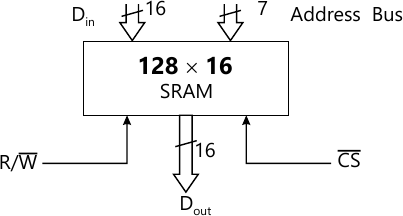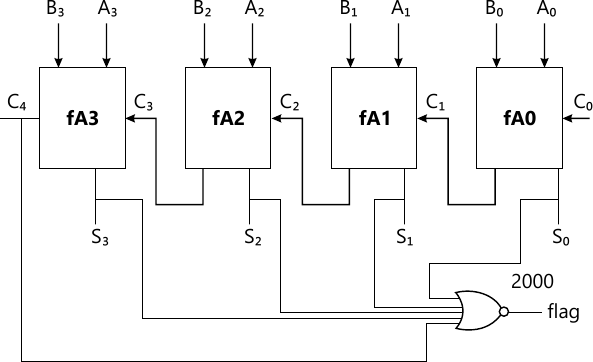MORE IN Fundamentals of HDL
Total marks: --
Total time: --
INSTRUCTIONS
(1) Assume appropriate data and state your reasons
(2) Marks are given to the right of every question
(3) Draw neat diagrams wherever necessary

1 (a) Name the different types of operations in HDL. Explain the bitwise, unary and Boolean logical operations present in Verilog with example.
8 M
1 (b) Name the VHDL data types. Explain the physical data type and composite data type in VHDL.
6 M
1 (c) Write the result of the following operation if A=10010011 and B=01101111:
i) A sr/04 ii) B) sla 03 iii) A <<02 iv) A%2 v) !(&B) vi) A & B in VHDL.
6 M

2 (a) Write the VHDL code for 2×2 unsigned combinational array multiplier using dataflow description.
6 M
2 (b) Write the Verilog description for 4 bit ripple carry adder. Assume 5ns delay for all the gates and description using dataflow.
8 M
2 (c) Explain how signal declaration is done in VHDL and verilog.
6 M

3 (a) With the help of booth algorithm multiply the number (-8)× (7). Also write the VHDL code to realize the same.
10 M
3 (b) Write an HDL code to realize the positive edge triggered JK flip flop.
i) Use if statement in VHDL
ii) Use case statement in Verilog HDL.
10 M

4 (a) Write the logic circuit for performing 3 bit comparison using 3 full adder. Also write the structural description to realize the same in VHDL.
10 M
4 (b) What is binding? Explain how binding between entity and architecture is done in VHDL and also binding between library and module in VHDL.
5 M
4 (c) Write the truth table of a logic system having 3 input and when the odd number of inputs are high then the output of the system will be high. Also write the Verilog code to realize the same using structural description.
5 M

5 (a) What is the need of procedure and task? Explain the declaration and body of the task.
4 M
5 (b) Write the procedure for converting an unsigned binary to an integer.
8 M
5 (c) What is a function? Write the code for finding greater of two signed numbers in Verilog using function.
8 M

6 (a) What is the need for mixed types description?
4 M
6 (b) Using package declaration declare one dimensional array type with N elements and L number of bits in each element. Write a VHDL code for finding the largest element present in one dimensional array declared using package.
8 M
6 (c) Write the truth table for the SRAM shown in Fig. Q6(c). Write a Verilog HDL code to read or write the data from SRAM.8 M

7 (a) Write the mixed language description of an adder shown in Fig. Q7(a). Invoke a VHDL full adder from Verilog.10 M
7 (b) Write the truth table of JK flipflop with clear. Describe the JK flipflop with clear using mixed language description.
10 M

8 (a) Write the general steps of synthesis in form of a flowchart and explain it.
10 M
8 (b) Write VHDL and Verilog code for signal assignment statement y=3x with x as of size bits. Also show the mapping of this signal assignment to gate level.
10 M

More question papers from Fundamentals of HDL